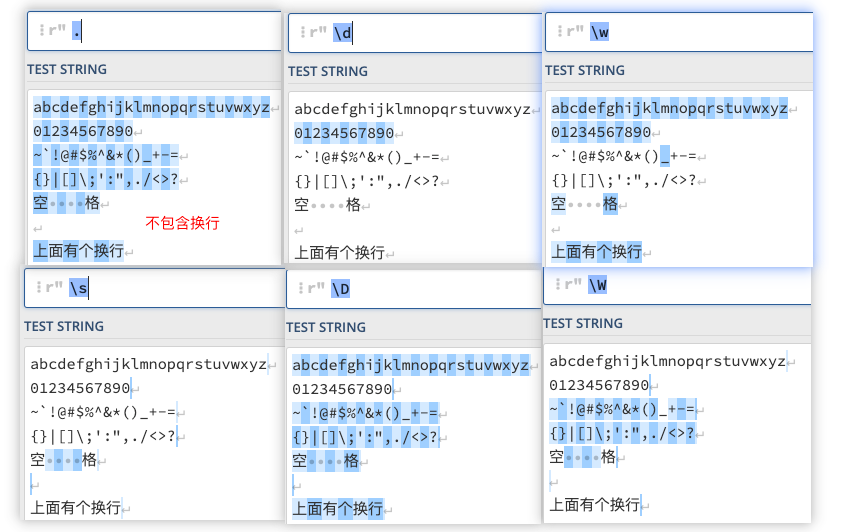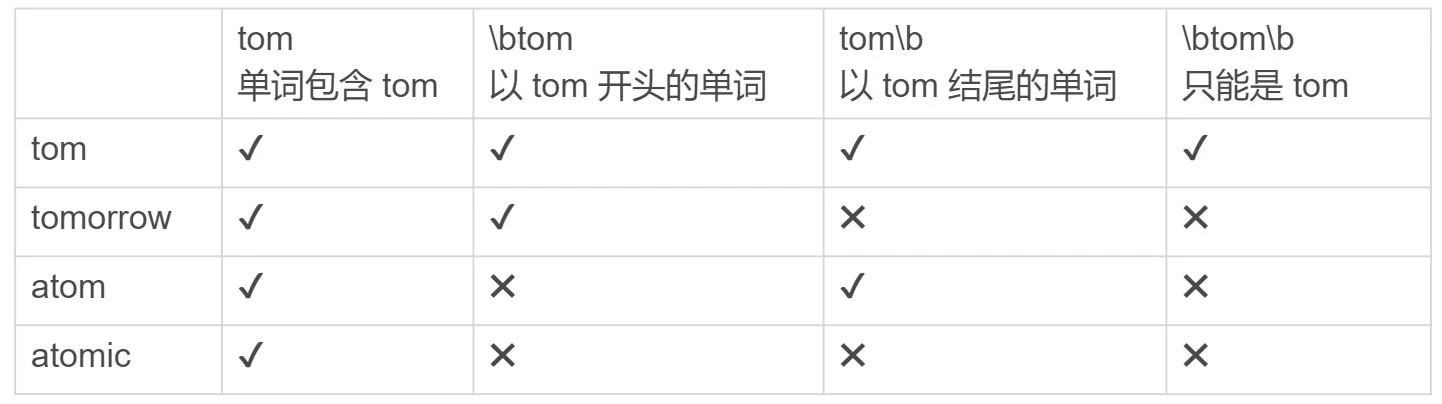# 正则表达式¶

• 校验数据的有效性，比如校验手机号或邮箱格式是否正确
• 查找符合要求的文本内容，比如查找文本中所有的数字
• 对文本进行切割替换等操作，比如将手机号中间四位替换为星号

• 最好要写注释，于人于己都是好事
• 能用普通字符串处理的，坚决用普通字符串处理
• 尽量将一个复杂的正则，分解为多个简单的正则

## 元字符¶

• `.` 除换行符外的任意字符（re.S模式下可以匹配换行符）
• `\s`（space） 任意空白符
• `\d`（digit） 任意数字，等同于`[0-9]`
• `\w`（word） 任意字母数字下划线（包括中文）• `{n}` n个
• `{m,n}` m～n个
• `{m,}` 最少m个
• `{,n}` 最多n个

• `*` >=0，即任意多个，等同于`{0,}`
• `+` >=1，即至少1个，正数，等同于`{1,}`
• `?` 0或1个，即至多1个，有或没有，等同于`{0,1}`

• `aa|bb|cc` 多字符二选一，比如：`https?|ftp:\/\/`
• `[abcde]` 单字符多选一，取反：`[^abcde]`
• `[a-z]` 连续字母多选一
• `[1-10]` 连续数字多选一
• `[0-9a-fA-F]` 可以匹配任意一个16进制的数字

## 边界¶• `^x` 拖字符，必须以x开头
• `x\$` 美元符，必须以x结尾

## 转义字符¶

• `\s` 任意空白符
• `\n` 换行，Windows平台的换行为`\r\n`
• `\r` 回车
• `\f` 换页
• `\t` 制表(水平)
• `\v` 制表(垂直)

## 匹配机制¶

• 贪婪匹配 Greedy，尽可能多的匹配，回溯，默认
• 非贪婪匹配 Lazy，尽可能少的匹配，回溯，加`?`
• 独占模式 Possessive，不回溯的贪婪匹配，匹配不上即失败，加`+`
``````import re

re.findall(r'a{1,3}', 'aaab')  # 贪婪模式，['aaa']
re.findall(r'a{1,3}?', 'aaab')  # 非贪婪模式，['a', 'a', 'a']
re.findall(r'a{1,3}+', 'aaab')  # 独占模式，内置的re库不支持会报错，re.error: multiple repeat
``````

## 匹配模式¶

• `(?i)` 不区分大小写模式 Case-Insensitive
• `(?s)` 点号通配，改变的是`.`号的匹配行为，可以匹配换行，也叫单行模式 Single Line
• `(?m)` 多行匹配模式，改变的是`^``\$`的匹配行为，可以匹配上每行的开头或结尾 Multiline
• `(?#comment)` 注释模式，可以在正则中添加注释

## 规范¶

• 基础正则(GNU BRE)，需要转义，比如：`grep``sed`
• 扩展正则(GNU ERE)，无需转义，比如：`awk`

`egrep`，或者`grep -E`可以使用扩展正则，`grep -P`可以使用PCRE正则

`sed -r`可以使用扩展正则

## Python中的正则表达式¶

``````import re

"""

1. pattern 正则表达式
2. string 要匹配的字符串
3. flags 匹配模式，多个可用竖线分割：re.I | re.S
------
re.I Case-Insensitive 忽略大小写
re.S Single Line 单行模式，点号可以匹配包括换行符在内的任意字符，在提取爬虫内容时常用
re.M Multiline 多行模式，影响^和\$
---
re.L Locale 本地化识别
re.A ASCII字符模式
re.U Unicode 根据Unicode字符集解析字符，影响 \w\W \b\B
re.X Verbose 忽略字符串中的空白、tab、换行符
"""

# 查找文本，仅返回开头符合规则的对象，匹配则返回re.Match对象，否则返回None
# match(pattern, string, flags=0)
obj = re.match(r"123", "abc123")  # None
obj = re.match(r"abc", "abc123")  # <re.Match object; span=(0, 3), match='abc'>
obj.group()  # "abc"
obj.span()  # (0,3)
obj.start()  # 0
obj.end()  # 3

# 查找文本，仅返回第一处符合规则的对象，其它与match方法相同
# search(pattern, string,flags=0)
obj = re.search(r"789", "abc123def456")  # None
obj = re.search(r"\d{3}", "abc123def456")  # <re.Match object; span=(3, 6), match='123'>
obj.group()  # "123"
obj.span()  # (3,6)

# 查找文本，list形式返回所有符合规则的字符
# findall(pattern, string, flags=0)
re.findall(r"\d{3}", "abc123def456")  # ['123', '456']

# 分割文本，list形式返回，类似字符串的.split()方法
# re.split(pattern, string, maxsplit=0, flags=0)
# 参数maxsplit用于指定分割次数
re.split(r"[\+\-\*\/]", "5+4*3-2/1")  # 从四则运算符处将文本分割：['5', '4', '3', '2', '1']
re.split(r"[\+\-\*\/]", "5+4*3-2/1", maxsplit=2)  # 从四则运算符处将文本分割2次：['5', '4', '3-2/1']
re.split(r"([\+\-\*\/])", "5+4*3-2/1")  # 从四则运算符处将文本分割，并保留运算符(使用正则分组功能实现)：['5', '+', '4', '*', '3', '-', '2', '/', '1']

# 替换文本，返回替换后的新文本，类似字符串的.replace()方法
# sub(pattern, repl, string, count=0, flags=0)
# 参数count用于指定替换次数，默认=0替换全部
# 参数repl可引用pattern，还可以是自定义函数
re.sub(r"l", "L", "Hello, World!")  # 'HeLLo, WorLd!'
re.sub(r"l", "L", "Hello, World!", count=2)  # 'HeLLo, World!'
re.sub(r"(World)", r"<em>\1<em>", "Hello, World!")  # 'Hello, <em>World<em>!'

# 将正则编译为对象，然后调用以上方法，实现复用提高效率
# compile(pattern, flags=0)
obj = re.compile(r"abc")
obj.match("abc123").group()  # "abc"
# 还可以返回迭代器对象，使用group()方法调用
iters = obj.finditer(string)
for i in iters:
print(i.group())
``````

## 常用示例¶

• `.*` 任意多个任意字符，通配
• `.+` 至少一个任意字符，匹配核心文本内容
• `\w+` 单词
``````# 提取所有单词，所有单词都是用引号隔开，且中文引号内视作一个单词
text = '''we found “the little cat” is in the hat, we like “the little cat”'''
regex = r'''\w+|“[^”]+“'''
re.findall(regex, text)  # ['we', 'found', '“the little cat”', 'is', 'in', 'the', 'hat', 'we', 'like', '“the little cat”']

# 去掉连续出现多次的重复单词
test_str = "the little cat cat is in the hat hat hat, we like it."
regex = r"(\w+)(\s\1)+"
subst = r"\1"
re.sub(regex, subst, test_str)  # "the little cat is in the hat, we like it."
``````